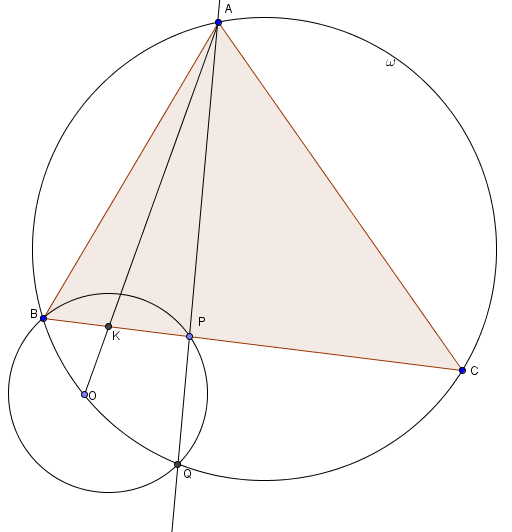# Circumcenter On Circumcircle

Geometry Level 4Let $\omega$ be the circumcircle of a triangle $\triangle ABC.$ A line $\ell$ passing through point $A$ intersects segment $BC$ and $\omega$ at points $P,Q$ respectively (where $Q \neq A$). Let $O$ be the circumcenter of $\triangle BPQ,$ and let $AO$ intersect $BC$ at $K.$ Given that $O$ lies on $\omega,$ find $\angle AKB$ in degrees.

Note: The image is not drawn to scale.

×